# Determine the magnitude of the resultant of the two forces and its direction, measured clockwisefrom the x-axis. (Use parallelogram law and law of sine and cosines) Given that F, = 500 N,F2 = 300 N, y = 30°, and a = 45°.УF2хF1

Question
10 views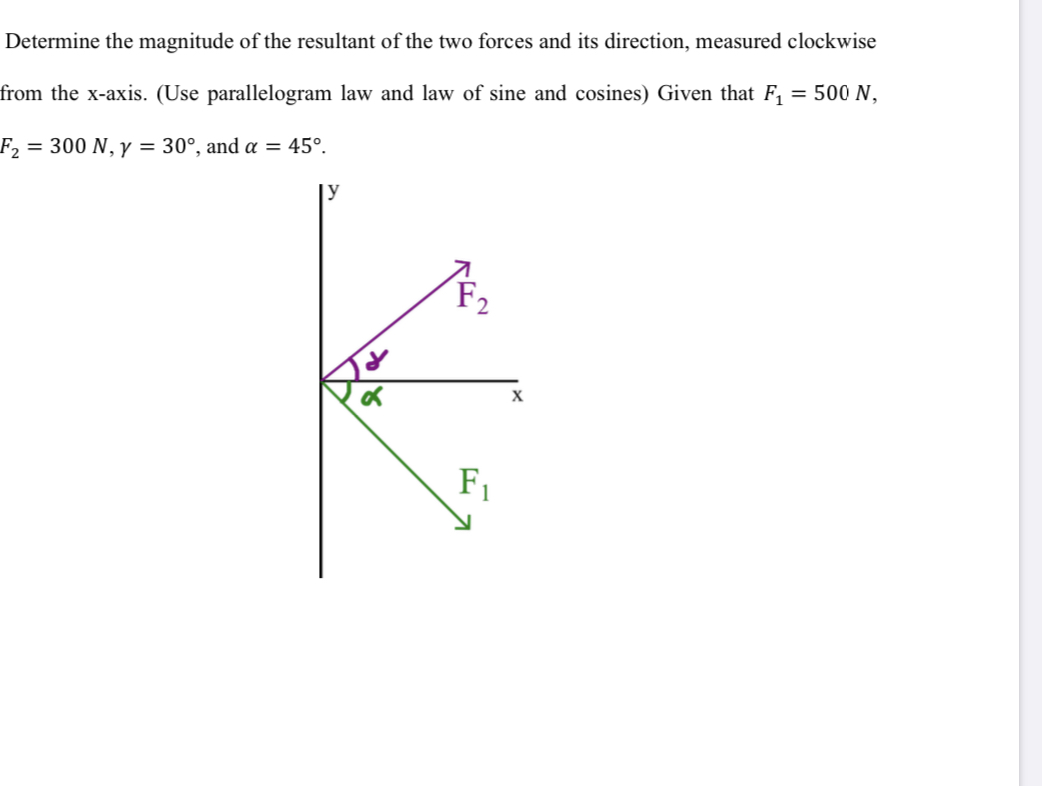help_outlineImage TranscriptioncloseDetermine the magnitude of the resultant of the two forces and its direction, measured clockwise from the x-axis. (Use parallelogram law and law of sine and cosines) Given that F, = 500 N, F2 = 300 N, y = 30°, and a = 45°. У F2 х F1 fullscreen
check_circle

Step 1

Drawing parallelogram for the given system of force –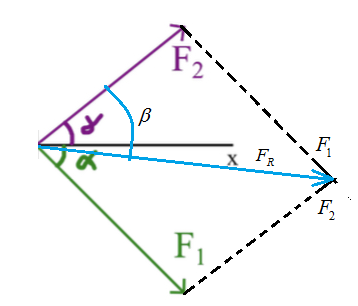Writing the resultant of two force formula from parallelogram law,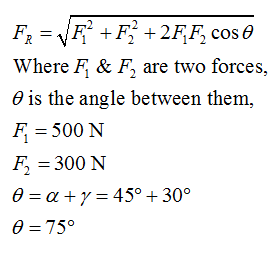Step 2

Plugging the values in resultant formula,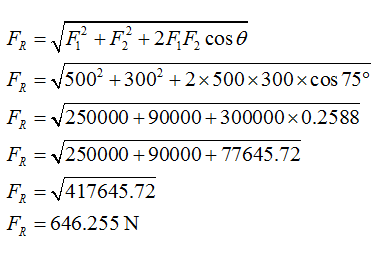Hence, the resultant force is 646.255 N.

Step 3

Calculating its direction by law of sine,

Let the resultant makes and angle of beta from F2 force,

Then from one triangle of a parallelogram, applying sine law,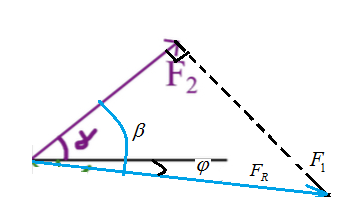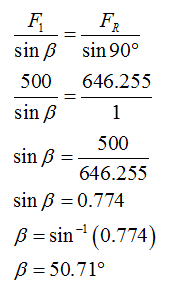...

### Want to see the full answer?

See Solution

#### Want to see this answer and more?

Solutions are written by subject experts who are available 24/7. Questions are typically answered within 1 hour.*

See Solution
*Response times may vary by subject and question.
Tagged in